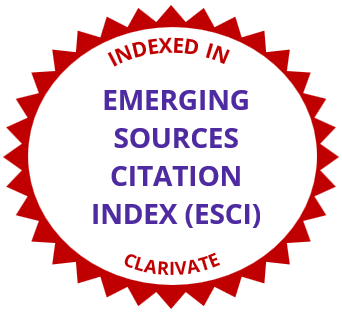# International Electronic Journal of Mathematics EducationGrade Twelve Students Establishing the Relationship Between Differentiation and Integration in Calculus Using graphs
• Article Type: Research Article
• International Electronic Journal of Mathematics Education, 2016 - Volume 11 Issue 9, pp. 3371-3385
• Published Online: 25 Nov 2016
• Article Views: 2102 | Article Download: 1622

# Abstract

Calculus is an important subject for science, engineering, and other fields of studies but phenomenally it is abstract and difficult to learn. Despite its importance, the teaching of introductory calculus always emphasizes manipulation of algebraic notations and rote learning. Most students learn the how instead of the why of calculus due to extensive use of algebraic symbols and notations. Therefore, graphing activities were developed based on the learning cycle approach and the lesson was taught for an hour in the classroom. This study investigates the effectiveness of the lesson in understanding the relationship between differentiation and integration calculus and to measure the students’ attitude towards the learning unit. Sixty-five grade twelve students from higher secondary school were selected for the study. The test scores showed that there was significance improvement in the post-test scores compared to the pre-test scores. It was also found that the lesson was effective and enriching.

# References

• Abraham, M. (1989). Research on instructional strategies. Journal of College Science Teaching, 18, 185–187.
• Gravemeijer, K., & Doorman, M. (1999). Context problems in realistic mathematics education: A calculus course as an example. Educational Studies in Mathematics, 39, 111–129.
• Hughes-Hallett, D., Gleason, A., Flath, D., Gordon, S., Lomen, D., Lovelock, D. Tecosky-Feldman, J. (2003). Calculus. NY: John Wiley and Sons, Inc.
• Kaput, J. (1994). Democratizing access to calculus: New routes to old roots. In A. Schoenfeld (Ed.), Mathematical thinking and problem solving (pp. 77–156). Hillsdale, NJ: Erlbaum.
• Karplus, R. (1980). Teaching for the development of reasoning. Research in Science Education, 10(1), 1–9.
• Lang, X. (1999). CAI and the reform of calculus education in China. International Journal of Mathematical Education in Science and Technology, 30(3), 399–404.
• Lawson, A. E., Abraham, M. R., & Renner, J. W. (1989). NARST monograph: Number one. A theory of instruction: Using the learning cycle to teach science concepts and thinking skills. Cincinnati, OH: National Association for Research in Science Teaching.
• Mundy, F., & Graham, K. (1994). Research in calculus learning: Understanding of limits, derivatives, and integrals. In E. Dubinsky (Ed.), Research issues in undergraduate mathematics learning: Preliminary analysis and results (pp. 31–45). Washington, DC: Mathematical Association of America.
• Orhun, N. (2012). Graphical understanding in Mathematics education: Derivative functions and students’ difficulties. Procedia-Social and Behavioral Sciences, 55, 679–684.
• Orton A.(1983a). Students’ understanding of differentiation. Educational Studies in Mathematics. 14(3), 235–50.
• Orton A. (1983b). Students’ understanding of integration. Educational Studies in Mathematics. 14(1), 1–18.
• Peterson, I. (1986). The troubled state of calculus. Science News, 129(14), 220–21.
• Piaget, J. (1952). The origins of intelligence in children (M. Cook, Trans.). New York, NY: William Warder Norton & Company.
• Ryan, J. (1992). Integrating computers into the teaching of calculus: Differentiating student needs. In B. Southwell, B. Perry & K. Owens (Eds), Proceedings of the 13th Annual Conference of the Mathematics Education Research Group of Australasia (pp. 478–487). University of Western Sydney, Richmond, 4–8 July.
• Sokolowski A, Yalvac B, Loving C. (2011). Science modelling in pre-calculus: how to make mathematics problems contextually meaningful. International Journal of Mathematical Education in Science and Technology. ; 42(3), 283–97.
• Steen, L. A. (1988). Introduction. In L. A. Steen (Ed.), Calculus for a new century: A pump, not a filter (pp. xi–xiii). Washington, DC: Mathematical Association of America.
• Sunal, D. W. (2007). The learning cycle: A comparison of models of strategies for conceptual reconstruction—A review of the literature. Retrieved from http://astlc.ua.edu/ScienceInElem&MiddleSchool/565LearningCycle-Comparing- Models.htm.
• Tall, D. (1982). Conceptual foundations of the calculus and the computer. The Proceedings of the fourth international conference on college mathematics teaching. 73–88.
• Tall, D. (1986). A graphical approach to integration and the fundamental theorem. Mathematics Teaching, 113, 48–51.